# RD Sharma Solutions for Class 11 Chapter 15 - Linear Inequations Exercise 15.1

In earlier classes, we have studied equations in one and two variables. An equation can be defined as a statement involving variables and a sign of equality. Similarly, inequation can be defined as a statement involving variables and a sign of inequality. In Exercise 15.1, we shall discuss problems on solving linear inequations in one variable. Students can understand the concept of inequations by using the solutions prepared by the tutors at BYJU’S. Solutions are provided with precision, in order to help students to sort their difficulties and reduce the fear of exams. The pdf of RD Sharma Class 11 Solutions is provided here, which students can easily download and start practising offline.

## Download the pdf of RD Sharma Solutions for Class 11 Maths Exercise 15.1 Chapter 15 – Linear Inequations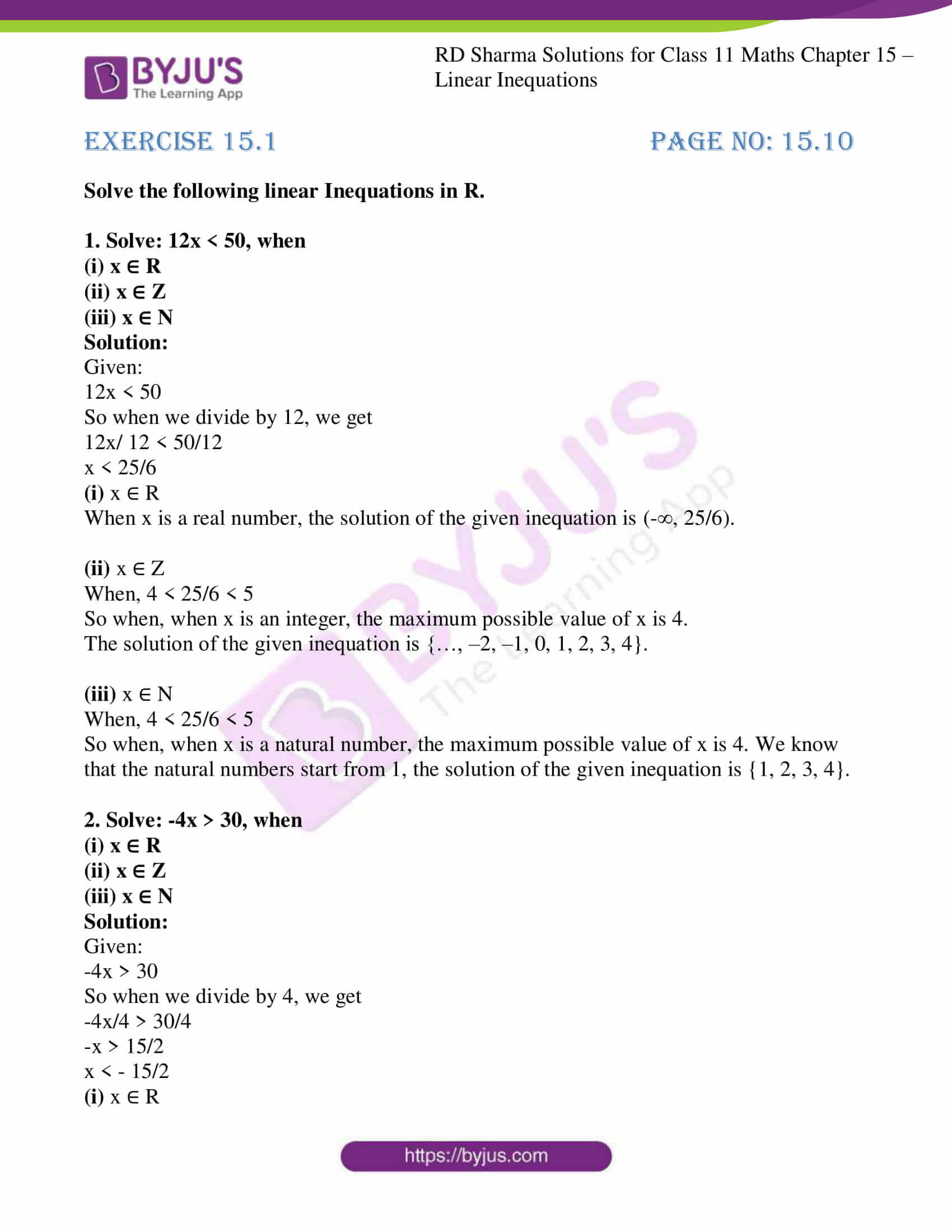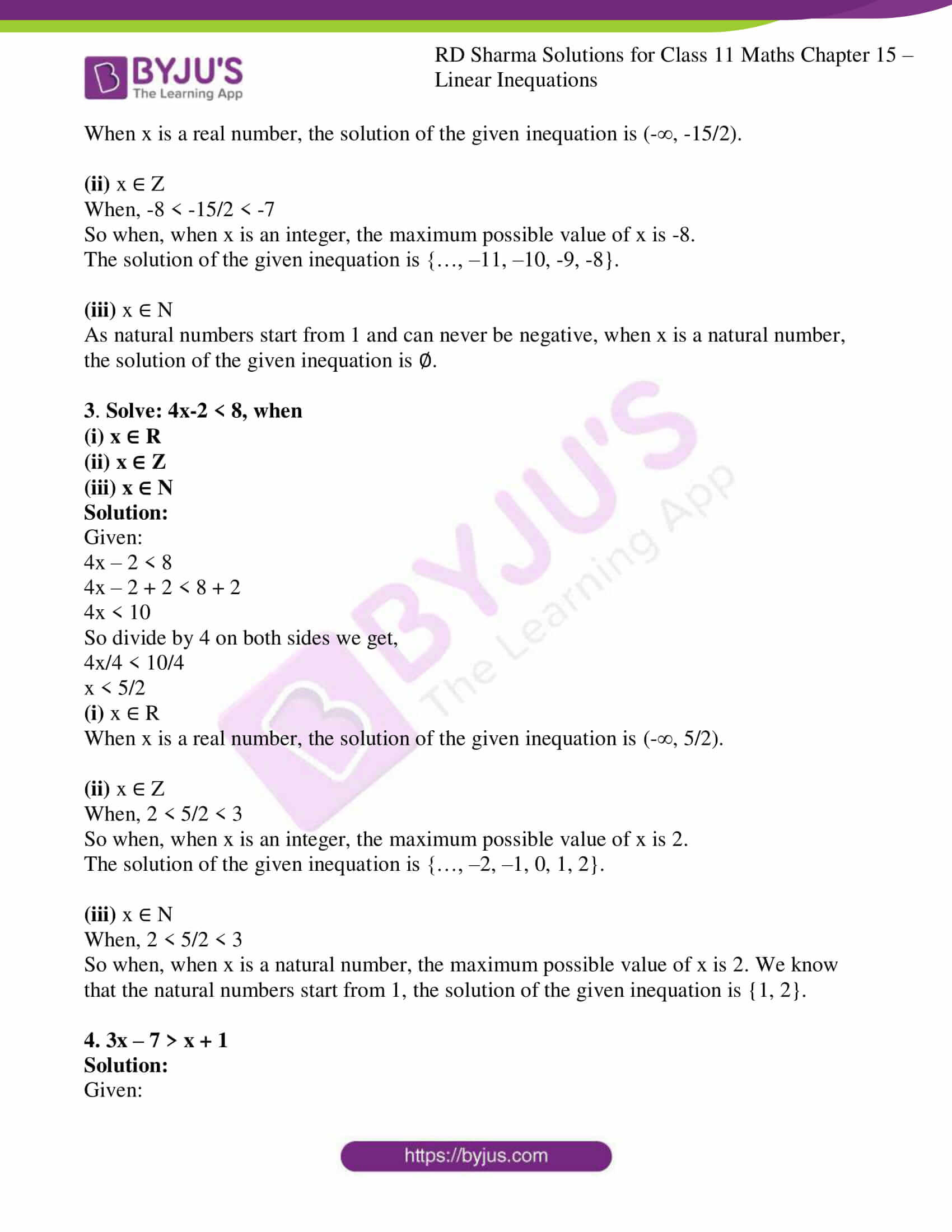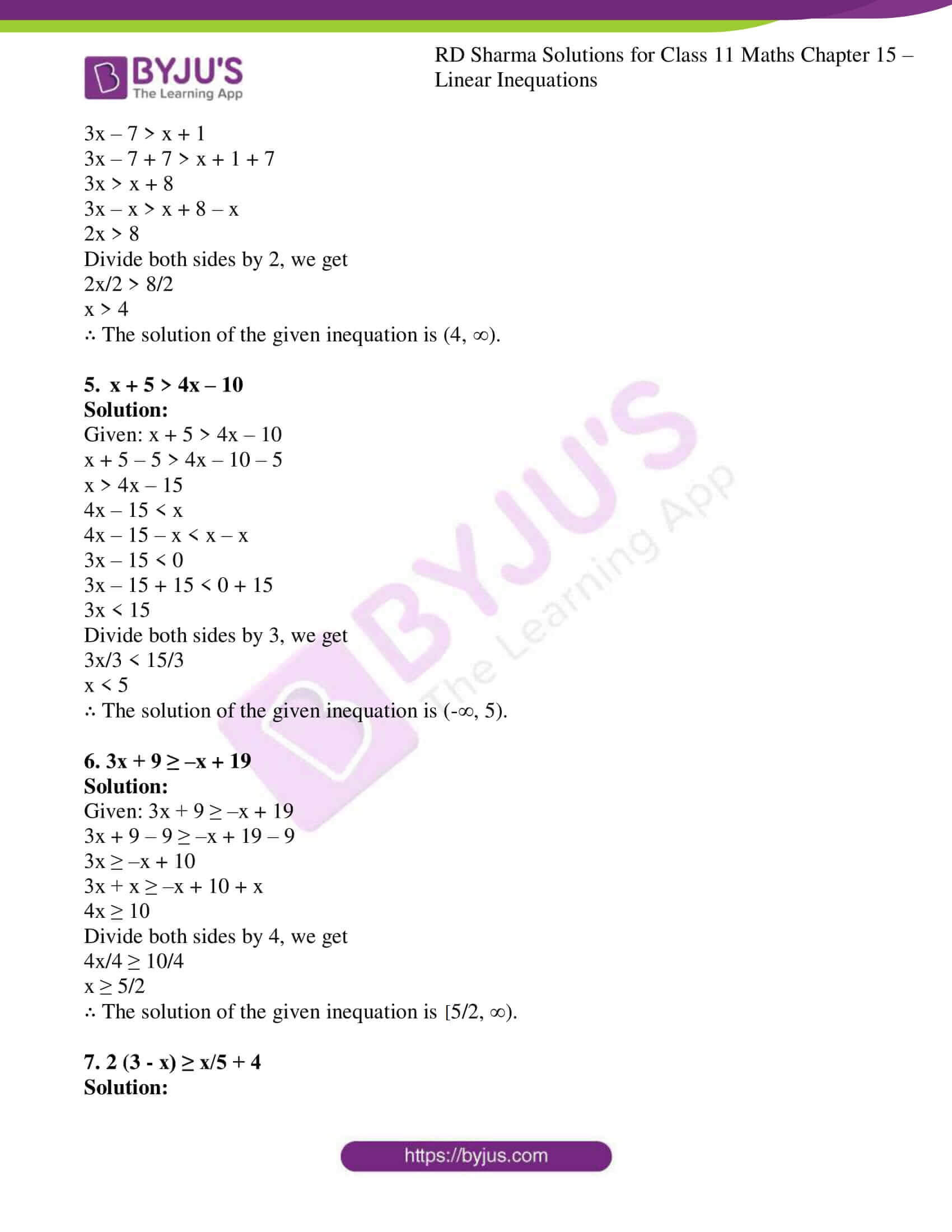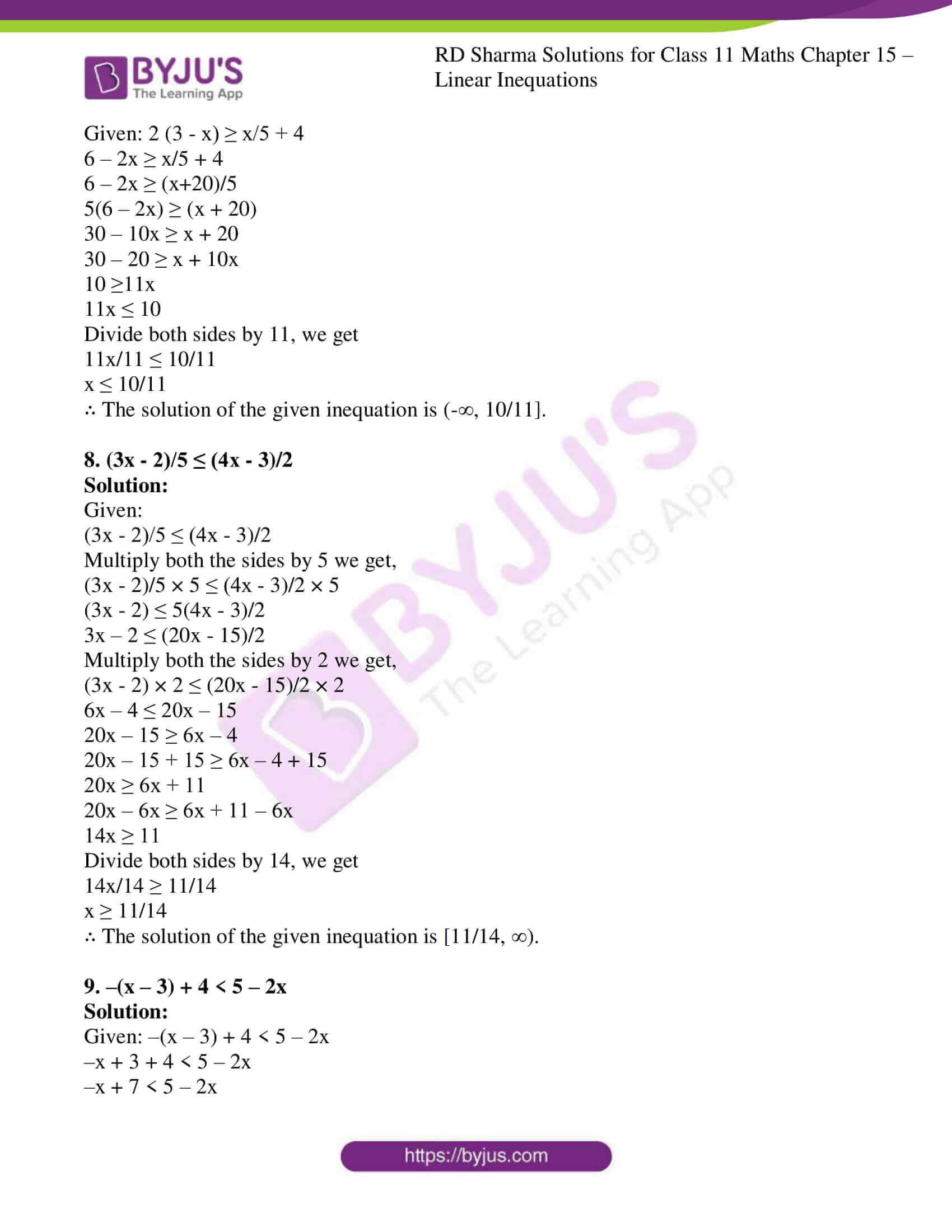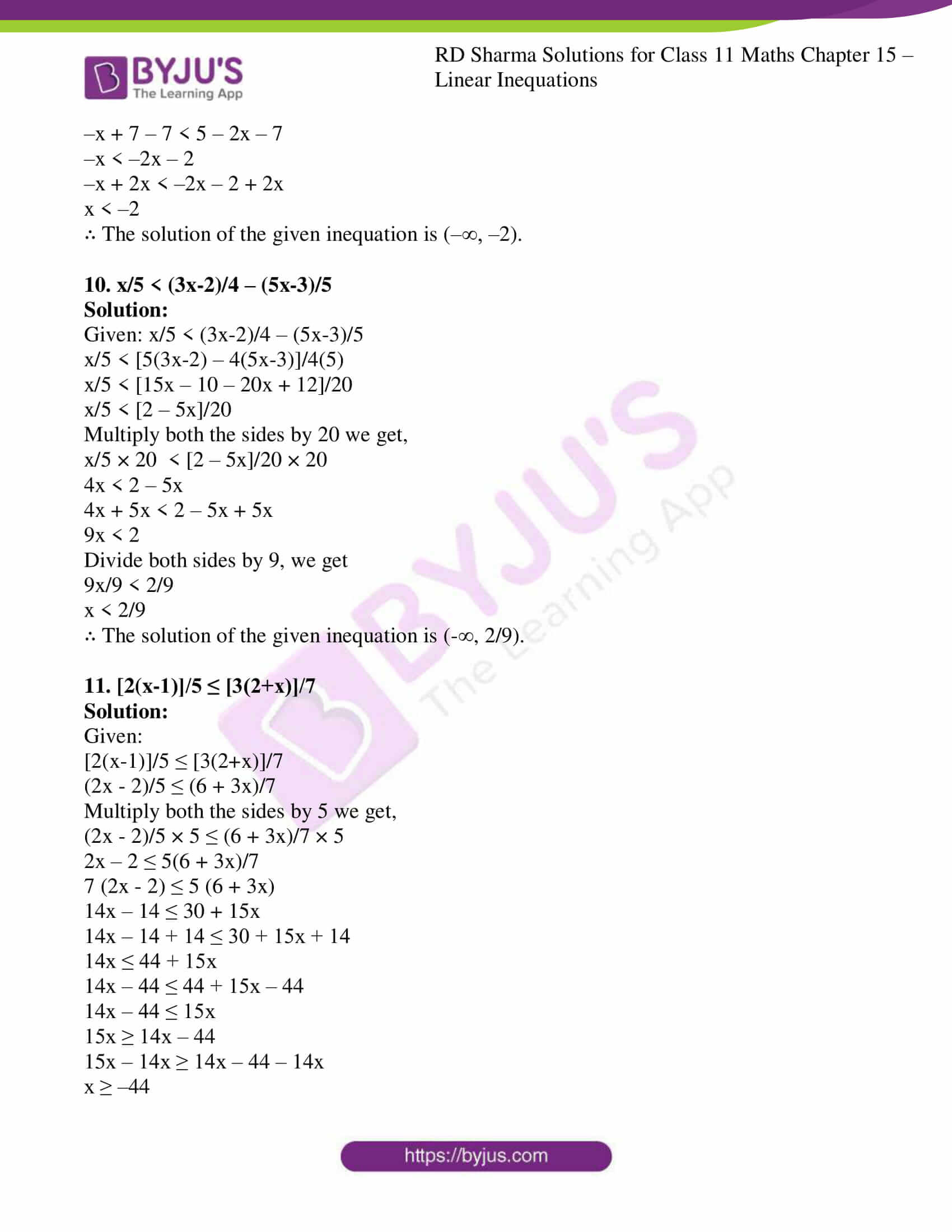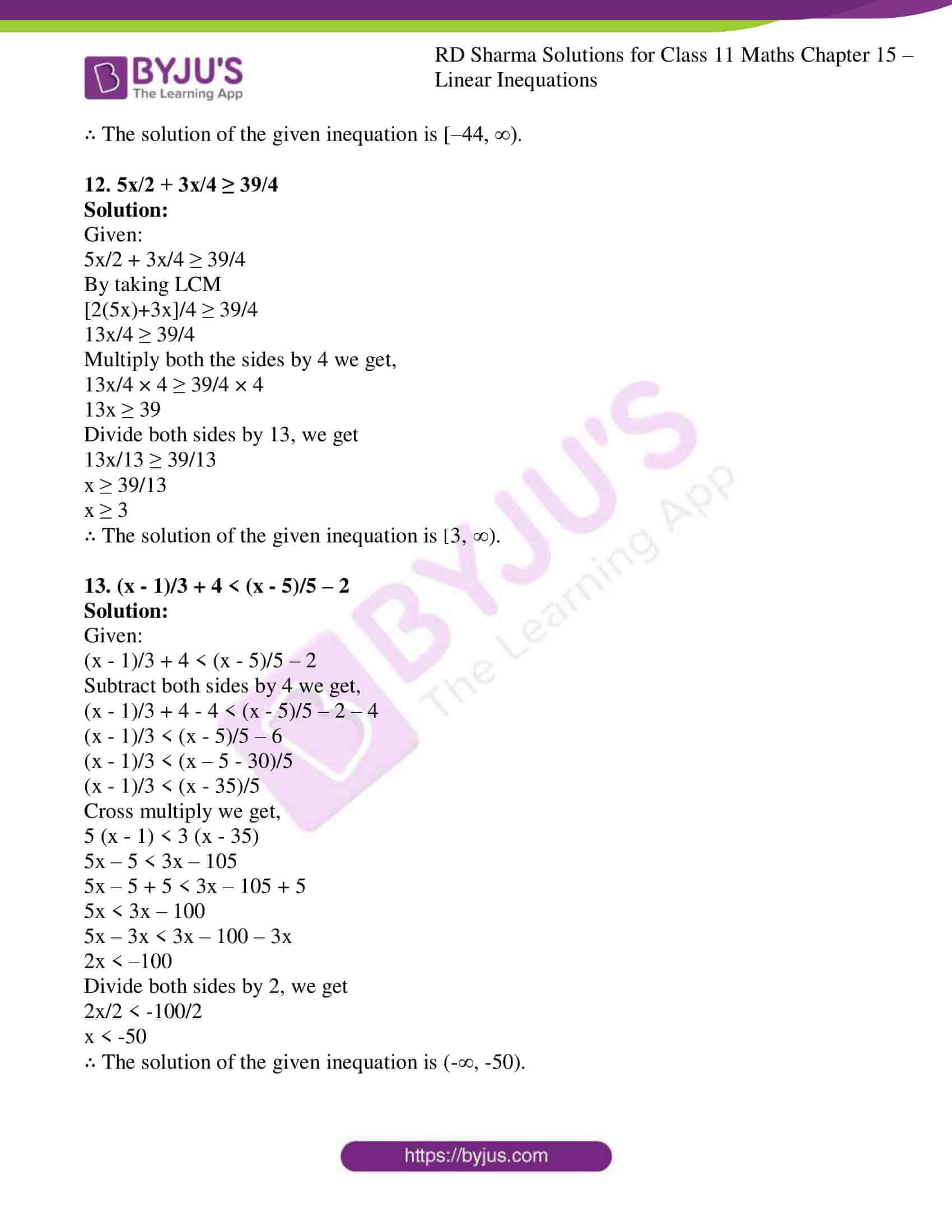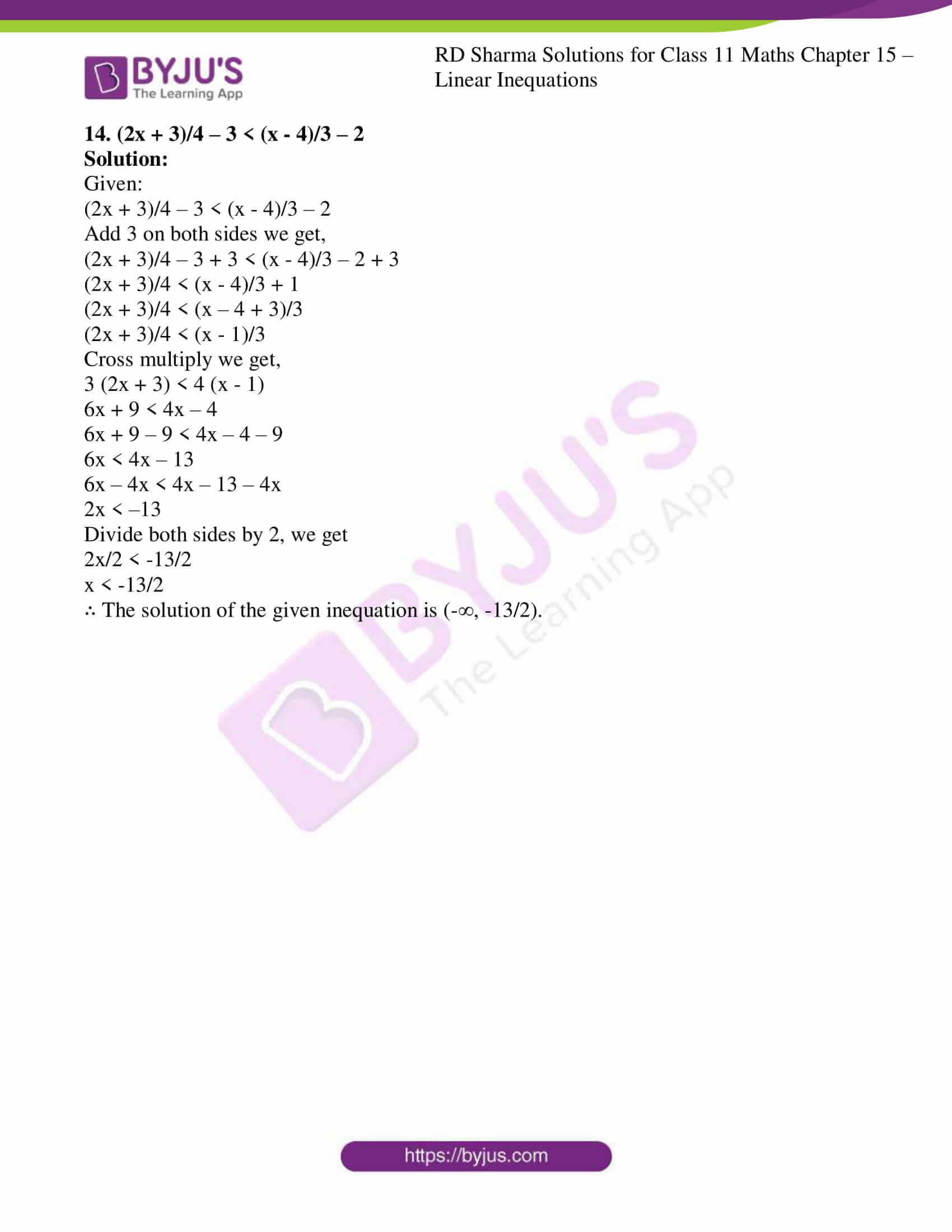### Also, access other exercises of RD Sharma Solutions for Class 11 Maths Chapter 15 – Linear Inequations

Exercise 15.2 Solutions

Exercise 15.3 Solutions

Exercise 15.4 Solutions

Exercise 15.5 Solutions

Exercise 15.6 Solutions

### Access answers to RD Sharma Solutions for Class 11 Maths Exercise 15.1 Chapter 15 – Linear Inequations

Solve the following linear Inequations in R.

1. Solve: 12x < 50, when

(i) x ∈ R

(ii) x ∈ Z

(iii) x ∈ N

Solution:

Given:

12x < 50

So when we divide by 12, we get

12x/ 12 < 50/12

x < 25/6

(i) x ∈ R

When x is a real number, the solution of the given inequation is (-∞, 25/6).

(ii) x ∈ Z

When, 4 < 25/6 < 5

So when, when x is an integer, the maximum possible value of x is 4.

The solution of the given inequation is {…, –2, –1, 0, 1, 2, 3, 4}.

(iii) x ∈ N

When, 4 < 25/6 < 5

So when, when x is a natural number, the maximum possible value of x is 4. We know that the natural numbers start from 1, the solution of the given inequation is {1, 2, 3, 4}.

2. Solve: -4x > 30, when

(i) x ∈ R

(ii) x ∈ Z

(iii) x ∈ N

Solution:

Given:

-4x > 30

So when we divide by 4, we get

-4x/4 > 30/4

-x > 15/2

x < – 15/2

(i) x ∈ R

When x is a real number, the solution of the given inequation is (-∞, -15/2).

(ii) x ∈ Z

When, -8 < -15/2 < -7

So when, when x is an integer, the maximum possible value of x is -8.

The solution of the given inequation is {…, –11, –10, -9, -8}.

(iii) x ∈ N

As natural numbers start from 1 and can never be negative, when x is a natural number, the solution of the given inequation is ∅.

3. Solve: 4x-2 < 8, when

(i) x ∈ R

(ii) x ∈ Z

(iii) x ∈ N

Solution:

Given:

4x – 2 < 8

4x – 2 + 2 < 8 + 2

4x < 10

So divide by 4 on both sides we get,

4x/4 < 10/4

x < 5/2

(i) x ∈ R

When x is a real number, the solution of the given inequation is (-∞, 5/2).

(ii) x ∈ Z

When, 2 < 5/2 < 3

So when, when x is an integer, the maximum possible value of x is 2.

The solution of the given inequation is {…, –2, –1, 0, 1, 2}.

(iii) x ∈ N

When, 2 < 5/2 < 3

So when, when x is a natural number, the maximum possible value of x is 2. We know that the natural numbers start from 1, the solution of the given inequation is {1, 2}.

4. 3x – 7 > x + 1

Solution:

Given:

3x – 7 > x + 1

3x – 7 + 7 > x + 1 + 7

3x > x + 8

3x – x > x + 8 – x

2x > 8

Divide both sides by 2, we get

2x/2 > 8/2

x > 4

∴ The solution of the given inequation is (4, ∞).

5. x + 5 > 4x – 10

Solution:

Given: x + 5 > 4x – 10

x + 5 – 5 > 4x – 10 – 5

x > 4x – 15

4x – 15 < x

4x – 15 – x < x – x

3x – 15 < 0

3x – 15 + 15 < 0 + 15

3x < 15

Divide both sides by 3, we get

3x/3 < 15/3

x < 5

∴ The solution of the given inequation is (-∞, 5).

6. 3x + 9 ≥ –x + 19

Solution:

Given: 3x + 9 ≥ –x + 19

3x + 9 – 9 ≥ –x + 19 – 9

3x ≥ –x + 10

3x + x ≥ –x + 10 + x

4x ≥ 10

Divide both sides by 4, we get

4x/4 ≥ 10/4

x ≥ 5/2

∴ The solution of the given inequation is [5/2, ∞).

7. 2 (3 – x) ≥ x/5 + 4

Solution:

Given: 2 (3 – x) ≥ x/5 + 4

6 – 2x ≥ x/5 + 4

6 – 2x ≥ (x+20)/5

5(6 – 2x) ≥ (x + 20)

30 – 10x ≥ x + 20

30 – 20 ≥ x + 10x

10 ≥11x

11x ≤ 10

Divide both sides by 11, we get

11x/11 ≤ 10/11

x ≤ 10/11

∴ The solution of the given inequation is (-∞, 10/11].

8. (3x – 2)/5 ≤ (4x – 3)/2

Solution:

Given:

(3x – 2)/5 ≤ (4x – 3)/2

Multiply both the sides by 5 we get,

(3x – 2)/5 × 5 ≤ (4x – 3)/2 × 5

(3x – 2) ≤ 5(4x – 3)/2

3x – 2 ≤ (20x – 15)/2

Multiply both the sides by 2 we get,

(3x – 2) × 2 ≤ (20x – 15)/2 × 2

6x – 4 ≤ 20x – 15

20x – 15 ≥ 6x – 4

20x – 15 + 15 ≥ 6x – 4 + 15

20x ≥ 6x + 11

20x – 6x ≥ 6x + 11 – 6x

14x ≥ 11

Divide both sides by 14, we get

14x/14 ≥ 11/14

x ≥ 11/14

∴ The solution of the given inequation is [11/14, ∞).

9. –(x – 3) + 4 < 5 – 2x

Solution:

Given: –(x – 3) + 4 < 5 – 2x

–x + 3 + 4 < 5 – 2x

–x + 7 < 5 – 2x

–x + 7 – 7 < 5 – 2x – 7

–x < –2x – 2

–x + 2x < –2x – 2 + 2x

x < –2

∴ The solution of the given inequation is (–∞, –2).

10. x/5 < (3x-2)/4 – (5x-3)/5

Solution:

Given: x/5 < (3x-2)/4 – (5x-3)/5

x/5 < [5(3x-2) – 4(5x-3)]/4(5)

x/5 < [15x – 10 – 20x + 12]/20

x/5 < [2 – 5x]/20

Multiply both the sides by 20 we get,

x/5 × 20 < [2 – 5x]/20 × 20

4x < 2 – 5x

4x + 5x < 2 – 5x + 5x

9x < 2

Divide both sides by 9, we get

9x/9 < 2/9

x < 2/9

∴ The solution of the given inequation is (-∞, 2/9).

11. [2(x-1)]/5 ≤ [3(2+x)]/7

Solution:

Given:

[2(x-1)]/5 ≤ [3(2+x)]/7

(2x – 2)/5 ≤ (6 + 3x)/7

Multiply both the sides by 5 we get,

(2x – 2)/5 × 5 ≤ (6 + 3x)/7 × 5

2x – 2 ≤ 5(6 + 3x)/7

7 (2x – 2) ≤ 5 (6 + 3x)

14x – 14 ≤ 30 + 15x

14x – 14 + 14 ≤ 30 + 15x + 14

14x ≤ 44 + 15x

14x – 44 ≤ 44 + 15x – 44

14x – 44 ≤ 15x

15x ≥ 14x – 44

15x – 14x ≥ 14x – 44 – 14x

x ≥ –44

∴ The solution of the given inequation is [–44, ∞).

12. 5x/2 + 3x/4 ≥ 39/4

Solution:

Given:

5x/2 + 3x/4 ≥ 39/4

By taking LCM

[2(5x)+3x]/4 ≥ 39/4

13x/4 ≥ 39/4

Multiply both the sides by 4 we get,

13x/4 × 4 ≥ 39/4 × 4

13x ≥ 39

Divide both sides by 13, we get

13x/13 ≥ 39/13

x ≥ 39/13

x ≥ 3

∴ The solution of the given inequation is [3, ∞).

13. (x – 1)/3 + 4 < (x – 5)/5 – 2

Solution:

Given:

(x – 1)/3 + 4 < (x – 5)/5 – 2

Subtract both sides by 4 we get,

(x – 1)/3 + 4 – 4 < (x – 5)/5 – 2 – 4

(x – 1)/3 < (x – 5)/5 – 6

(x – 1)/3 < (x – 5 – 30)/5

(x – 1)/3 < (x – 35)/5

Cross multiply we get,

5 (x – 1) < 3 (x – 35)

5x – 5 < 3x – 105

5x – 5 + 5 < 3x – 105 + 5

5x < 3x – 100

5x – 3x < 3x – 100 – 3x

2x < –100

Divide both sides by 2, we get

2x/2 < -100/2

x < -50

∴ The solution of the given inequation is (-∞, -50).

14. (2x + 3)/4 – 3 < (x – 4)/3 – 2

Solution:

Given:

(2x + 3)/4 – 3 < (x – 4)/3 – 2

Add 3 on both sides we get,

(2x + 3)/4 – 3 + 3 < (x – 4)/3 – 2 + 3

(2x + 3)/4 < (x – 4)/3 + 1

(2x + 3)/4 < (x – 4 + 3)/3

(2x + 3)/4 < (x – 1)/3

Cross multiply we get,

3 (2x + 3) < 4 (x – 1)

6x + 9 < 4x – 4

6x + 9 – 9 < 4x – 4 – 9

6x < 4x – 13

6x – 4x < 4x – 13 – 4x

2x < –13

Divide both sides by 2, we get

2x/2 < -13/2

x < -13/2

∴ The solution of the given inequation is (-∞, -13/2).